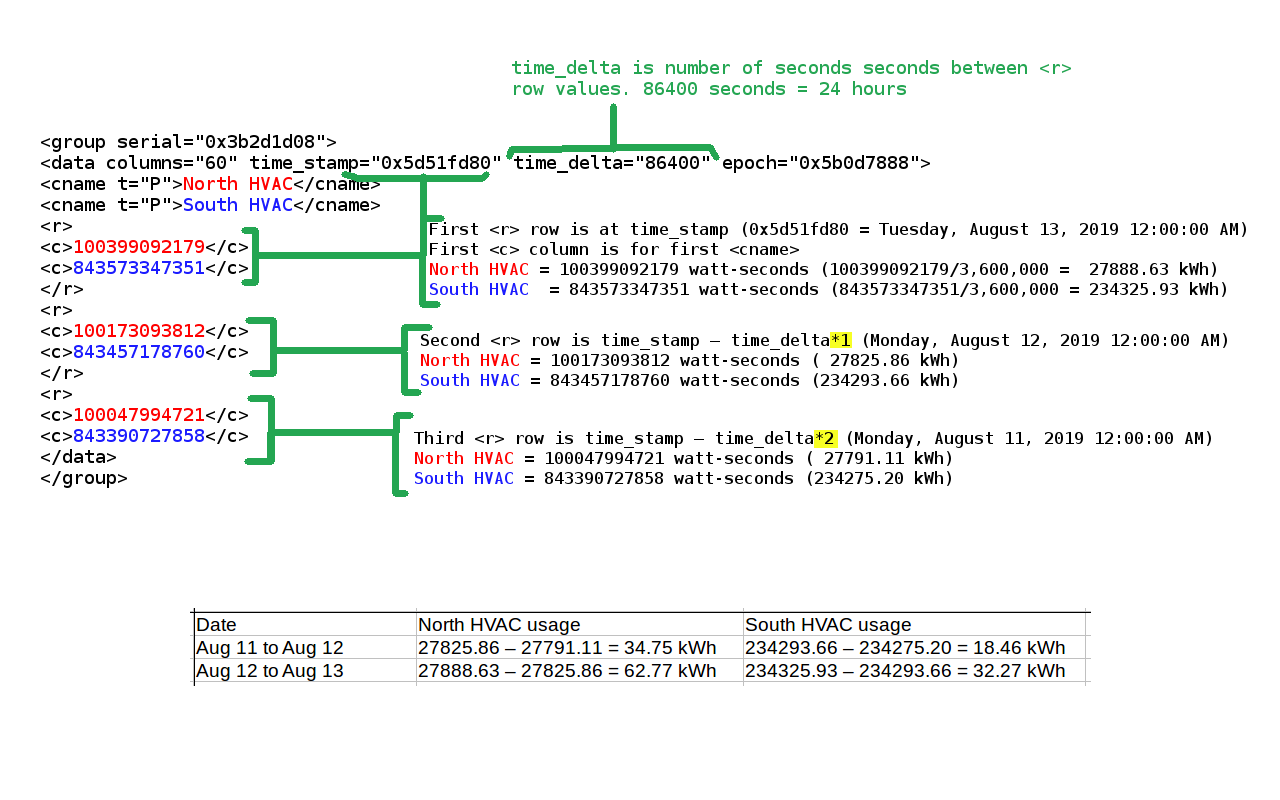# General Information

At the bottom of the page is the output of a minute granular export showing the past ten minutes of cumulative data (obtained with the URL http://DEVNAME.egaug.es/cgi-bin/egauge-show?m&n=10). time_stamp represents the time of the export (unix timestamp in hex); time_delta indicates the time in seconds between exports; epoch represents the date and time recording started on that device (unix timestamp in hex).

Each <cname> tag contains data on a column header; in this case, that translates to a physical register on the device. t indicates the register type (P for power, V for Voltage, etc) and the text inside the tag represents the register name as recorded on the device. Virtual registers may also be shows in the appropriate parameter is passed in the initial request.

Each row (<r> tag) contains a series of columns (<c> tag) that show the cumulative value of each register. To determine the average value of a register over a given time, simply find the difference between the two cumulative values and divide by the appropriate time delta. The resulting values are expressed in units based on the register type (see the chart in section 2.2.1 of the XML API document for the unit type).  It should be noted that prior to firmware 3.01 cumulative values do not necessarily count up from zero. On firmware 3.01 and newer passing the option when requesting data returns values relative to device epoch (ie, values start at zero). Using the parameter epoch in a data push has the same effect. This assumes that the date and time recording started option is set correctly on the eGauge.

Some examples (based on the sample output below):

Average Grid usage over the most recent minute 55357226851 - 55357243343 = −16492 / 60 = -274.86 Watts (remember, Power registers are bidirectional)

Average Voltage L2 over ten minutes 4511385868513 - 4511319123106 = 66745407 / 600 = 111242.345 mV / 1000 = 111.24 Volts

```<group serial="0x4e842294">
<data columns="12" time_stamp="0x564cb0e8" time_delta="60" epoch="0x55973268">
<cname t="P">Grid</cname>
<cname t="S">Grid*</cname>
<cname t="V">VL2</cname>
<cname t="V">VL1</cname>
<cname t="F">Frequency</cname>
<r>
<c>55357226851</c>
<c>7375247726</c>
<c>4511385868513</c>
<c>4528987513211</c>
<c>2217532746128</c>
</r>
<r>
<c>55357243343</c>
<c>7375223338</c>
<c>4511378482617</c>
<c>4528980146863</c>
<c>2217529147760</c>
</r>
<r>
<c>55357259861</c>
<c>7375198952</c>
<c>4511371100578</c>
<c>4528972784417</c>
<c>2217525549473</c>
</r>
<r>
<c>55357276431</c>
<c>7375174516</c>
<c>4511363715094</c>
<c>4528965418400</c>
<c>2217521950920</c>
</r>
<r>
<c>55357293137</c>
<c>7375149735</c>
<c>4511356276347</c>
<c>4528957999802</c>
<c>2217518352640</c>
</r>
<r>
<c>55357309872</c>
<c>7375124940</c>
<c>4511348838707</c>
<c>4528950580365</c>
<c>2217514754150</c>
</r>
<r>
<c>55357326630</c>
<c>7375100152</c>
<c>4511341406162</c>
<c>4528943162279</c>
<c>2217511155334</c>
</r>
<r>
<c>55357343410</c>
<c>7375075359</c>
<c>4511333976595</c>
<c>4528935743683</c>
<c>2217507556120</c>
</r>
<r>
<c>55357360207</c>
<c>7375050569</c>
<c>4511326550844</c>
<c>4528928327670</c>
<c>2217503956798</c>
</r>
<r>
<c>55357377048</c>
<c>7375025739</c>
<c>4511319123106</c>
<c>4528920909766</c>
<c>2217500357151</c>
</r>
</data>
</group>```

# Example: Energy and power for specific dates

You can make CGI calls to http://DEV-URL/cgi-bin/egauge-show where DEV-URL is the URL of your eGauge (for example, http://egaugehq.d.egauge.net/).

The T parameter lets you request data from specific points in time. It expects a comma separated list of Unix time-stamps.

The E parameter requests the values be relative to the Date and Time when recording started. This needs to be set correctly in Settings -> Date & time when recording started. It effectively makes the reading start at zero when the date and time when recording started is set to, otherwise the raw database value could be arbitrary. This requires firmware v3.02 or greater.

The a parameter requests total and virtual registers, such as "Usage" and "Generation". This is optional.

http://egaugehq.d.egauge.net/cgi-bin/egauge-show?a&E&T=1514764800,1483228800 returns data for January 1 2018 00:00:00 UTC, and January 1 2017 00:00:00 UTC respectively, using epoch-relative values and requesting total and virtual registers. The output below has all the other registers except for Usage and Generation removed for readability.

```<group serial="0x3b2d1cb7">
<data columns="27" time_stamp="0x5a497a00" time_delta="60" epoch="0x52a0f760">
<cname t="P">use</cname>
<cname t="P">gen</cname>
<r>
<c>241517238757</c>
<c>0</c>
</r>
</data>
<data time_stamp="0x58684680" time_delta="900">
<r>
<c>171138633823</c>
<c>0</c>
</r>
</data>
</group>```

Generation is zero because there is none recorded on this device.

Usage for timestamp 0x5a497a00 (Jan 1 2018) is 241517238757 joules (241517238757/3600000 =  67088 kWh).

Usage for timestamp 0x58684680 (Jan 1 2017) is 171138633823 joules (171138633823/3600000 = 47538 kWh).

If you want power instead of energy, subtract the values and divide by the amount of time between them:

67088 kWh - 47538 kWh = 19550 kWh were used between 2017 and 2018. 1 year is 8760 hours, so 19550 kWh / 8760 h = 2.23 kW average over the year. This can be done using any two points in time.

Another description example: